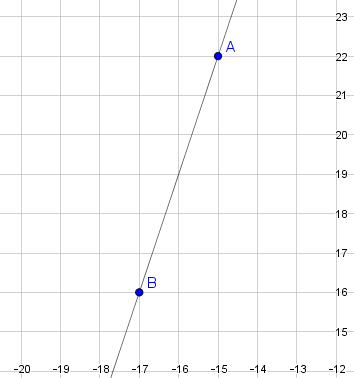# Linear Relationships

## Objective

Write linear equations using two given points on the line.

## Common Core Standards

### Core Standards

?

• 8.EE.B.6 — Use similar triangles to explain why the slope m is the same between any two distinct points on a non-vertical line in the coordinate plane; derive the equation y = mx for a line through the origin and the equation y = mx + b for a line intercepting the vertical axis at b.

• 8.F.B.4 — Construct a function to model a linear relationship between two quantities. Determine the rate of change and initial value of the function from a description of a relationship or from two (x, y) values, including reading these from a table or from a graph. Interpret the rate of change and initial value of a linear function in terms of the situation it models, and in terms of its graph or a table of values.

## Criteria for Success

?

1. Find the slope from two points on a line.
2. Use slope and a point on a line to determine where the line passes through the $y$-axis.
3. Write a linear equation in the form $y=mx+b$ using slope and $y$-intercept.
4. Write a linear equation for a word problem given information representing two ordered pairs.

## Tips for Teachers

?

Lessons 11 and 12 address writing linear equations using information about the line or situation. In Lesson 12, students are given two points on the line or two solutions to a situation in order to determine the slope or rate of change and the $y$-intercept or initial value.

#### Fishtank Plus

• Problem Set
• Student Handout Editor
• Vocabulary Package

## Anchor Problems

?

### Problem 1

A line is shown in the coordinate plane below.What is the equation for the line shown?

### Problem 2

A line passes through the points (4, 3) and (9, -7).

What is the equation for this line in slope-intercept form?

### Problem 3

A model airplane reaches a maximum altitude and then begins to descend at a constant rate in feet per second. After falling for 12 seconds, the model airplane has an altitude of 212 feet. After falling for 25 seconds, the model airplane’s altitude is 95 feet.

What function represents the altitude of the model airplane, $y$, after $x$ seconds of falling?

## Problem Set

?The following resources include problems and activities aligned to the objective of the lesson that can be used to create your own problem set.

?

### Problem 1

Tickets to a concert are available for early access on a special website. The website charges a fixed fee for early access to the tickets, and the tickets to the concert all cost the same amount with no additional tax. A friend of yours purchases 4 tickets on the website for a total of $162. Another friend purchases 7 tickets on the website for$270.

What function represents the total cost, $y$, for the purchase of $x$ tickets on the website?

Write an equation in slope-intercept form for the line that passes through the points ${(-2, -3)}$  and ${(1, 12)}$.